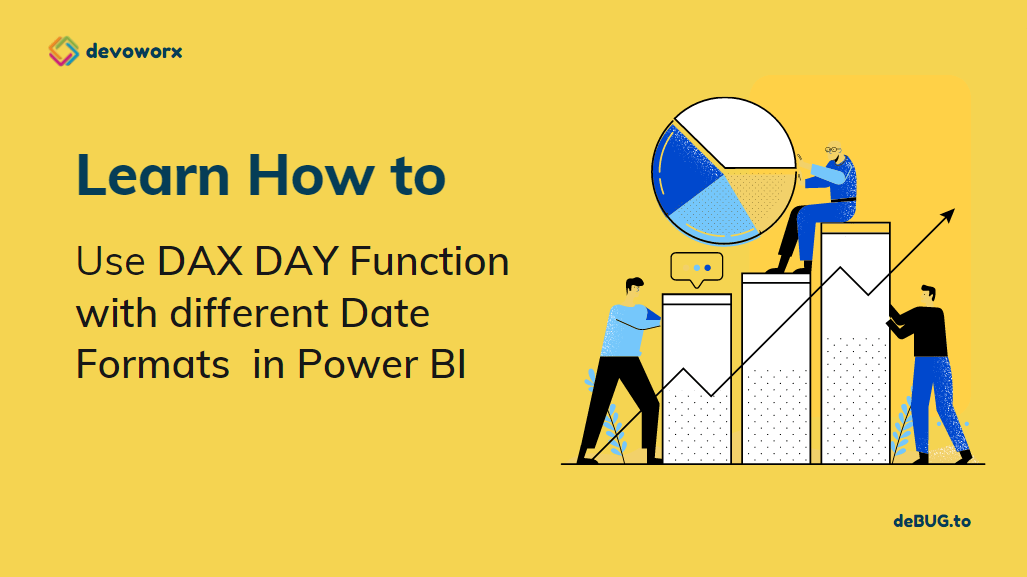## How to use DAX DAY Function in Power BI?

In this post, we will learn all you need to know about the DAX DAY function in Power BI as one of the Date time functions provided by DAX in Power BI. Also, we will show how the DAX handles the date values in DateTime format and returns the day of the month in Power BI.

## What is DAX in Power BI?

Data Analysis Expressions (DAX) is a formula expression language explicitly designed for the management of data models, the DAX formulas include functions and operators that can be used to create formulas and expressions in Analysis Services, Power BI, and Power Pivot in Excel.

DAX in Power BI includes a library of over 200 functions, operators, that are used by report designers to perform data analysis and calculations.

## What is DAX DAY Function?

The DAX DAY function extracts or returns a number from 1 to 31 that represents the day of the month from the given date.

• The DAY Function takes the date of the day you are trying to find.
• IT takes a date in datetime format
• Also the date argument can be provided to the DAY function by using another date function “by using an expression that returns a date”.
• You can specify Date as one of the following:
• An output of another date function.
• An expression that returns a date.
• A date in a datetime format.
• A date as text representation in one of the accepted string formats for dates.
• The DAY function uses the locale and date/time settings of the client computer when the date argument is a text representation of the date. For example If the current date time settings represent dates in the format of Month/Day/Year, then the string, “1/9/2022” is understood as a datetime value equivalent to 9th January, 2022 and the DAY function returns 8.

The DAY function will be Gregorian values regardless of the display format for the supplied date value. If date is Hijri, the returned values for the DAY function will be values associated with the equivalent Gregorian date

### DAX DAY Function Syntax

``DAY(<date>)``
• The DAX DAY function takes a date in DateTime format or a text representation of a date.
• Returns an integer indicating the day of the month according to the provided date argument.

### How to use DAX DAY Function in Power BI?

In this section, we’re going to provide two examples to learn how to use DAX DAY Function in Power BI.

#### Example 1

This example helps you to get the day of the month from a string date in Power BI.

Note: The Day function takes a date of “month-day-year” format, the DAX handles the date values in DateTime format and returns the day.

##### Steps
1. In Power BI Desktop, select which table you want to create your measure.
2. At “Home” tab, click on “New Measure”.
1. Write the below fromula to get the Day of the month for the provided date.
``Day of the Month = DAY("3-4-2022") it will return 4``
1. The output should look like below

You can also use the DAY function with a Date argument of the format “month/day/year”

``Day of the Month = DAY ("7/6/2022") // it will returns 6.``

### Example 2

In this example, the DAX DAY function takes the date of a format “day-month name” and, the DAX handles the date values in DateTime format and returns 12 for the day.

``Day of the Month = = DAY ("12-Jan")  // it will return 12``

### Example 3

In this example, the DAX DAY function takes a date with this format “month name/day/year” to return the day value.

``Day of the Month = DAY ("March 3, 2022") // it will returns 3``

## Using Today() in Power BI

In Power BI, you can use the TODAY() function to return the current date. so you can also use the DAY function as an output of another TODAY() function to return the day of today

``day of today = DAY (TODAY ()) it will return the day of today``

Note: you can also use NOW() function to get the current date and time in Power BI

## Getting the day from a date column in Power BI

In the previous sections, we learned how to get the day of the date whether the date was provided directly as a string in a different format or it was passed by a function like TODAY() and NOW().

Besides that, you can also get the day of a date from another date column in a table in Power BI as the following

### Steps

1. In Power BI Desktop, Click on Data Mode.
1. From the left side, Click on the table that you need to add a new column.
2. In “Table Tools” tab, click on “New Column” to create a new Column using DAX.
1. Write the below fromula to get the current day of the specifified column
``Day ot the month = DAY('Sales'[SalesDate]) ``
1. You can also use the below formula that returns the same result
``Day ot the month = 'Sales'[SalesDate].[Day]``
1. Go back to “Report View” to add a new table visual.
2. In values, add the new created column and other columns as you prefer to get the below result.

#### Conclusion

In conclusion, we have learned how to use the DAX DAY function in Power BI to return the day of the month of the provided date.